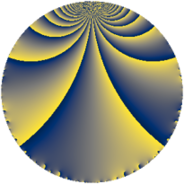# Properties

 Label 7728.2.a.biLevel $7728$ Weight $2$ Character orbit 7728.a Self dual yes Analytic conductor $61.708$ Analytic rank $0$ Dimension $2$ CM no Inner twists $1$

# Related objects

## Newspace parameters

 Level: $$N$$ $$=$$ $$7728 = 2^{4} \cdot 3 \cdot 7 \cdot 23$$ Weight: $$k$$ $$=$$ $$2$$ Character orbit: $$[\chi]$$ $$=$$ 7728.a (trivial)

## Newform invariants

 Self dual: yes Analytic conductor: $$61.7083906820$$ Analytic rank: $$0$$ Dimension: $$2$$ Coefficient field: $$\Q(\sqrt{41})$$ Defining polynomial: $$x^{2} - x - 10$$ Coefficient ring: $$\Z[a_1, \ldots, a_{11}]$$ Coefficient ring index: $$1$$ Twist minimal: no (minimal twist has level 3864) Fricke sign: $$-1$$ Sato-Tate group: $\mathrm{SU}(2)$

## $q$-expansion

Coefficients of the $$q$$-expansion are expressed in terms of $$\beta = \frac{1}{2}(1 + \sqrt{41})$$. We also show the integral $$q$$-expansion of the trace form.

 $$f(q)$$ $$=$$ $$q + q^{3} - q^{7} + q^{9} +O(q^{10})$$ $$q + q^{3} - q^{7} + q^{9} + \beta q^{11} + 2 q^{13} + ( -2 + 2 \beta ) q^{17} + ( 4 + \beta ) q^{19} - q^{21} - q^{23} -5 q^{25} + q^{27} + 2 q^{29} + ( 4 - 2 \beta ) q^{31} + \beta q^{33} + ( -4 + 2 \beta ) q^{37} + 2 q^{39} -\beta q^{41} + ( -6 + 2 \beta ) q^{43} + ( 10 - \beta ) q^{47} + q^{49} + ( -2 + 2 \beta ) q^{51} + ( 2 - 3 \beta ) q^{53} + ( 4 + \beta ) q^{57} + ( 6 - 3 \beta ) q^{59} + ( 10 - \beta ) q^{61} - q^{63} + ( 6 + 2 \beta ) q^{67} - q^{69} + ( 2 - 2 \beta ) q^{73} -5 q^{75} -\beta q^{77} + ( -4 - 2 \beta ) q^{79} + q^{81} + 10 q^{83} + 2 q^{87} -2 q^{89} -2 q^{91} + ( 4 - 2 \beta ) q^{93} + 6 q^{97} + \beta q^{99} +O(q^{100})$$ $$\operatorname{Tr}(f)(q)$$ $$=$$ $$2q + 2q^{3} - 2q^{7} + 2q^{9} + O(q^{10})$$ $$2q + 2q^{3} - 2q^{7} + 2q^{9} + q^{11} + 4q^{13} - 2q^{17} + 9q^{19} - 2q^{21} - 2q^{23} - 10q^{25} + 2q^{27} + 4q^{29} + 6q^{31} + q^{33} - 6q^{37} + 4q^{39} - q^{41} - 10q^{43} + 19q^{47} + 2q^{49} - 2q^{51} + q^{53} + 9q^{57} + 9q^{59} + 19q^{61} - 2q^{63} + 14q^{67} - 2q^{69} + 2q^{73} - 10q^{75} - q^{77} - 10q^{79} + 2q^{81} + 20q^{83} + 4q^{87} - 4q^{89} - 4q^{91} + 6q^{93} + 12q^{97} + q^{99} + O(q^{100})$$

## Embeddings

For each embedding $$\iota_m$$ of the coefficient field, the values $$\iota_m(a_n)$$ are shown below.

For more information on an embedded modular form you can click on its label.

Label $$\iota_m(\nu)$$ $$a_{2}$$ $$a_{3}$$ $$a_{4}$$ $$a_{5}$$ $$a_{6}$$ $$a_{7}$$ $$a_{8}$$ $$a_{9}$$ $$a_{10}$$
1.1
 −2.70156 3.70156
0 1.00000 0 0 0 −1.00000 0 1.00000 0
1.2 0 1.00000 0 0 0 −1.00000 0 1.00000 0
 $$n$$: e.g. 2-40 or 990-1000 Significant digits: Format: Complex embeddings Normalized embeddings Satake parameters Satake angles

## Atkin-Lehner signs

$$p$$ Sign
$$2$$ $$1$$
$$3$$ $$-1$$
$$7$$ $$1$$
$$23$$ $$1$$

## Inner twists

This newform does not admit any (nontrivial) inner twists.

## Twists

By twisting character orbit
Char Parity Ord Mult Type Twist Min Dim
1.a even 1 1 trivial 7728.2.a.bi 2
4.b odd 2 1 3864.2.a.g 2

By twisted newform orbit
Twist Min Dim Char Parity Ord Mult Type
3864.2.a.g 2 4.b odd 2 1
7728.2.a.bi 2 1.a even 1 1 trivial

## Hecke kernels

This newform subspace can be constructed as the intersection of the kernels of the following linear operators acting on $$S_{2}^{\mathrm{new}}(\Gamma_0(7728))$$:

 $$T_{5}$$ $$T_{11}^{2} - T_{11} - 10$$ $$T_{13} - 2$$ $$T_{17}^{2} + 2 T_{17} - 40$$

## Hecke characteristic polynomials

$p$ $F_p(T)$
$2$ $$T^{2}$$
$3$ $$( -1 + T )^{2}$$
$5$ $$T^{2}$$
$7$ $$( 1 + T )^{2}$$
$11$ $$-10 - T + T^{2}$$
$13$ $$( -2 + T )^{2}$$
$17$ $$-40 + 2 T + T^{2}$$
$19$ $$10 - 9 T + T^{2}$$
$23$ $$( 1 + T )^{2}$$
$29$ $$( -2 + T )^{2}$$
$31$ $$-32 - 6 T + T^{2}$$
$37$ $$-32 + 6 T + T^{2}$$
$41$ $$-10 + T + T^{2}$$
$43$ $$-16 + 10 T + T^{2}$$
$47$ $$80 - 19 T + T^{2}$$
$53$ $$-92 - T + T^{2}$$
$59$ $$-72 - 9 T + T^{2}$$
$61$ $$80 - 19 T + T^{2}$$
$67$ $$8 - 14 T + T^{2}$$
$71$ $$T^{2}$$
$73$ $$-40 - 2 T + T^{2}$$
$79$ $$-16 + 10 T + T^{2}$$
$83$ $$( -10 + T )^{2}$$
$89$ $$( 2 + T )^{2}$$
$97$ $$( -6 + T )^{2}$$